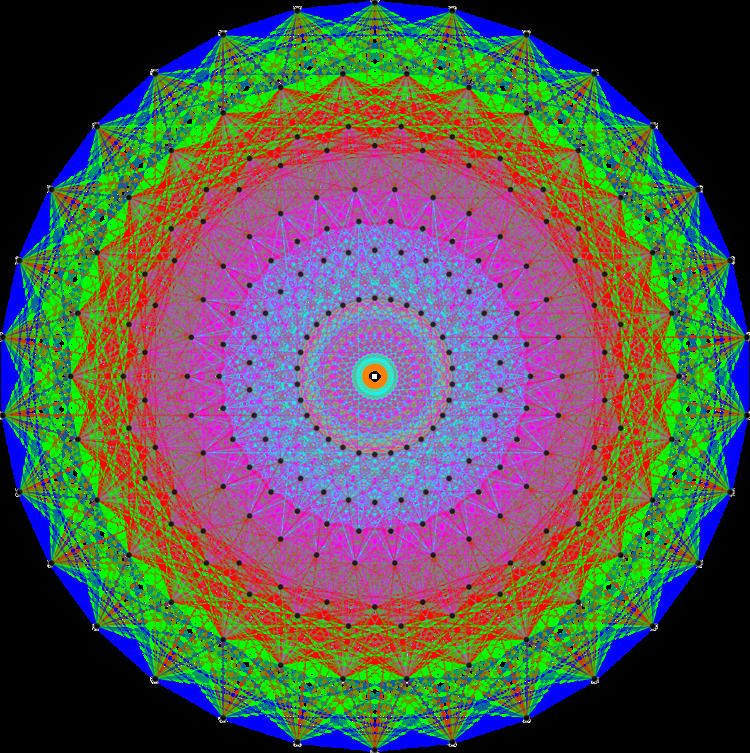# Representation theory of the Poincaré group

Updated on
Covid-19In mathematics, the representation theory of the Poincaré group is an example of the representation theory of a Lie group that is neither a compact group nor a semisimple group. It is fundamental in theoretical physics.

In a physical theory having Minkowski space as the underlying spacetime, the space of physical states is typically a representation of the Poincaré group. (More generally, it may be a projective representation, which amounts to a representation of the double cover of the group.)

In a classical field theory, the physical states are sections of a Poincaré-equivariant vector bundle over Minkowski space. The equivariance condition means that the group acts on the total space of the vector bundle, and the projection to Minkowski space is an equivariant map. Therefore, the Poincaré group also acts on the space of sections. Representations arising in this way (and their subquotients) are called covariant field representations, and are not usually unitary.

For a discussion of such unitary representations, see Wigner's classification.

In quantum mechanics, the state of the system is determined by the Schrödinger equation, which is invariant under Galilean transformations. Quantum field theory is the relativistic extension of quantum mechanics, where relativistic (Lorentz/Poincaré invariant) wave equations are solved, "quantized", and act on a Hilbert space composed of Fock states; eigenstates of the theory's Hamiltonian which are states with a definite number of particles with individual 4-momentum. There are no finite unitary representations of the full Lorentz (and thus Poincaré) transformations due to the non-compact nature of Lorentz boosts (rotations in Minkowski space along a space and time axis).

In case of spin 1/2 particles, it is possible to find a construction that includes both a finite-dimensional representation and a scalar product preserved by this representation by associating a 4-component Dirac spinor ψ with each particle. These spinors transform under Lorentz transformations generated by the gamma matrices ( γ μ ). It can be shown that the scalar product

ψ | ϕ = ψ ¯ ϕ = ψ γ 0 ϕ

is preserved. It is not, however, positive definite, so the representation is not unitary.

## References

Similar Topics
Song of the Sarong
John Bach
Waldo Ponce
Topics